Скачать презентацию Chapter 7 — Rate of Return Analysis Click

4b2e2a9410eaef610c86f0d91767bae2.ppt

• Количество слайдов: 20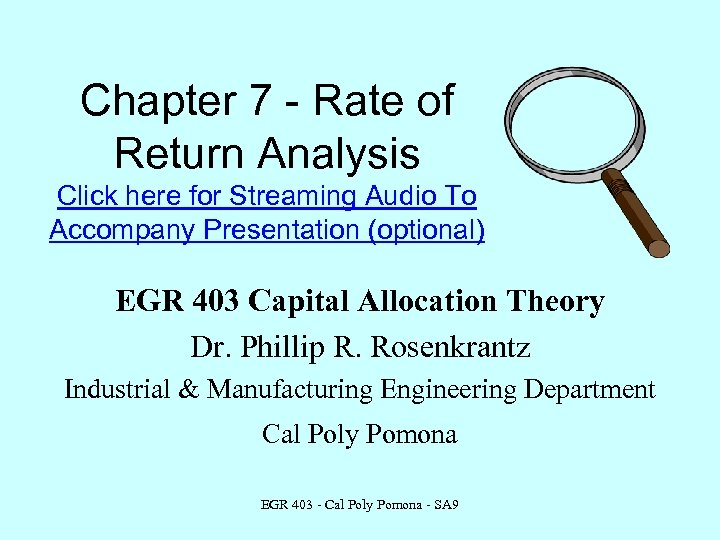Chapter 7 - Rate of Return Analysis Click here for Streaming Audio To Accompany Presentation (optional) EGR 403 Capital Allocation Theory Dr. Phillip R. Rosenkrantz Industrial & Manufacturing Engineering Department Cal Poly Pomona EGR 403 - Cal Poly Pomona - SA 9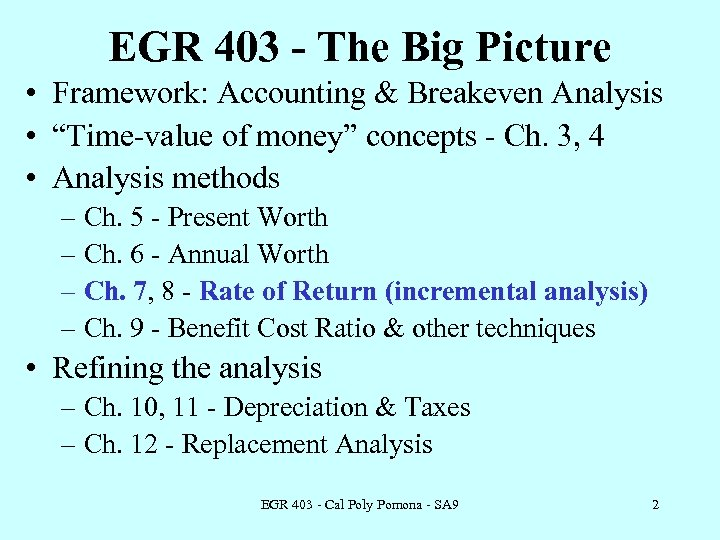EGR 403 - The Big Picture • Framework: Accounting & Breakeven Analysis • “Time-value of money” concepts - Ch. 3, 4 • Analysis methods – Ch. 5 - Present Worth – Ch. 6 - Annual Worth – Ch. 7, 8 - Rate of Return (incremental analysis) – Ch. 9 - Benefit Cost Ratio & other techniques • Refining the analysis – Ch. 10, 11 - Depreciation & Taxes – Ch. 12 - Replacement Analysis EGR 403 - Cal Poly Pomona - SA 9 2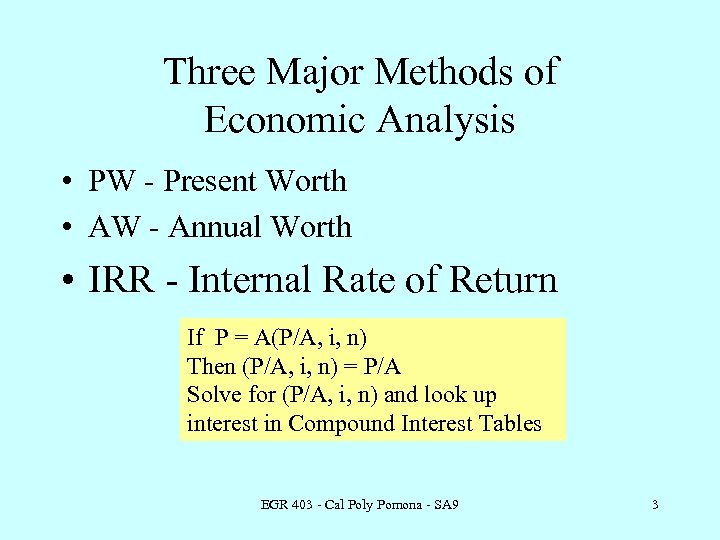Three Major Methods of Economic Analysis • PW - Present Worth • AW - Annual Worth • IRR - Internal Rate of Return If P = A(P/A, i, n) Then (P/A, i, n) = P/A Solve for (P/A, i, n) and look up interest in Compound Interest Tables EGR 403 - Cal Poly Pomona - SA 9 3Internal Rate of Return (IRR) • The interest rate paid on the unpaid balance of a loan such that the payment schedule makes the unpaid loan balance equal to zero when the final payment is made. Ex: P = \$5000, i = 10%, n = 5 EGR 403 - Cal Poly Pomona - SA 9 4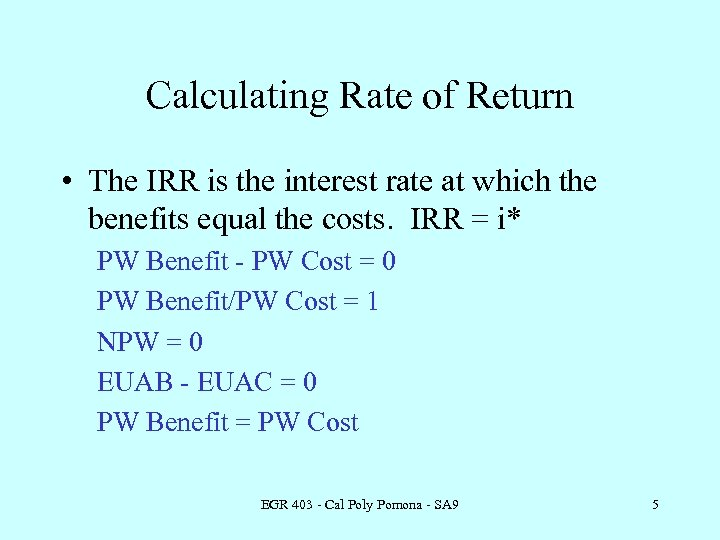Calculating Rate of Return • The IRR is the interest rate at which the benefits equal the costs. IRR = i* PW Benefit - PW Cost = 0 PW Benefit/PW Cost = 1 NPW = 0 EUAB - EUAC = 0 PW Benefit = PW Cost EGR 403 - Cal Poly Pomona - SA 9 5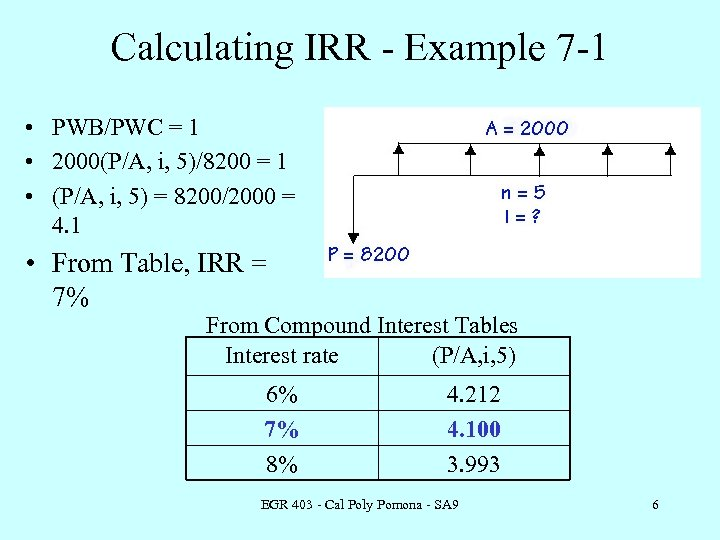Calculating IRR - Example 7 -1 • PWB/PWC = 1 • 2000(P/A, i, 5)/8200 = 1 • (P/A, i, 5) = 8200/2000 = 4. 1 • From Table, IRR = 7% From Compound Interest Tables Interest rate (P/A, i, 5) 6% 7% 8% 4. 212 4. 100 3. 993 EGR 403 - Cal Poly Pomona - SA 9 6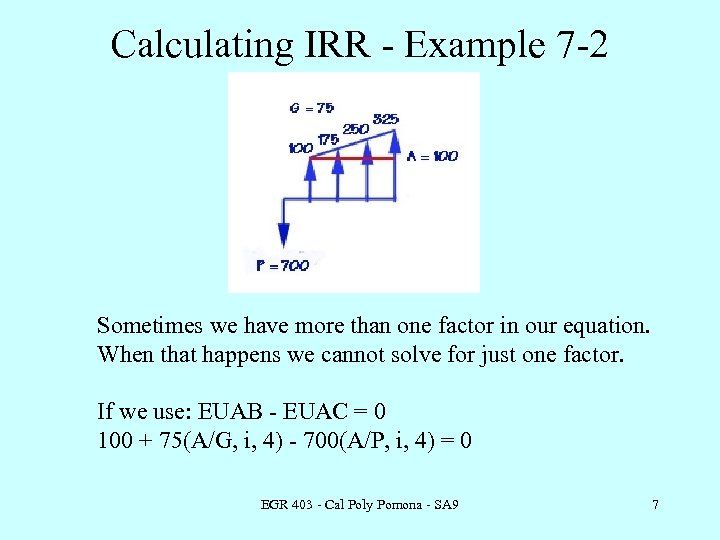Calculating IRR - Example 7 -2 Sometimes we have more than one factor in our equation. When that happens we cannot solve for just one factor. If we use: EUAB - EUAC = 0 100 + 75(A/G, i, 4) - 700(A/P, i, 4) = 0 EGR 403 - Cal Poly Pomona - SA 9 7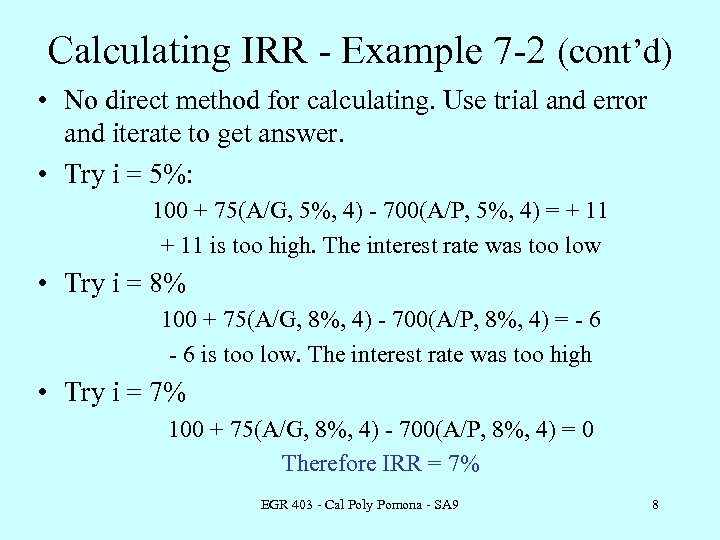Calculating IRR - Example 7 -2 (cont’d) • No direct method for calculating. Use trial and error and iterate to get answer. • Try i = 5%: 100 + 75(A/G, 5%, 4) - 700(A/P, 5%, 4) = + 11 is too high. The interest rate was too low • Try i = 8% 100 + 75(A/G, 8%, 4) - 700(A/P, 8%, 4) = - 6 is too low. The interest rate was too high • Try i = 7% 100 + 75(A/G, 8%, 4) - 700(A/P, 8%, 4) = 0 Therefore IRR = 7% EGR 403 - Cal Poly Pomona - SA 9 8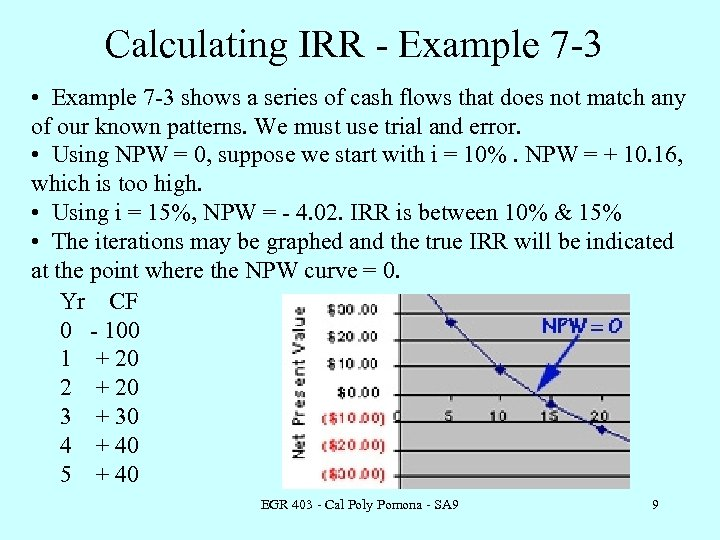Calculating IRR - Example 7 -3 • Example 7 -3 shows a series of cash flows that does not match any of our known patterns. We must use trial and error. • Using NPW = 0, suppose we start with i = 10%. NPW = + 10. 16, which is too high. • Using i = 15%, NPW = - 4. 02. IRR is between 10% & 15% • The iterations may be graphed and the true IRR will be indicated at the point where the NPW curve = 0. Yr CF 0 - 100 1 + 20 2 + 20 3 + 30 4 + 40 5 + 40 EGR 403 - Cal Poly Pomona - SA 9 9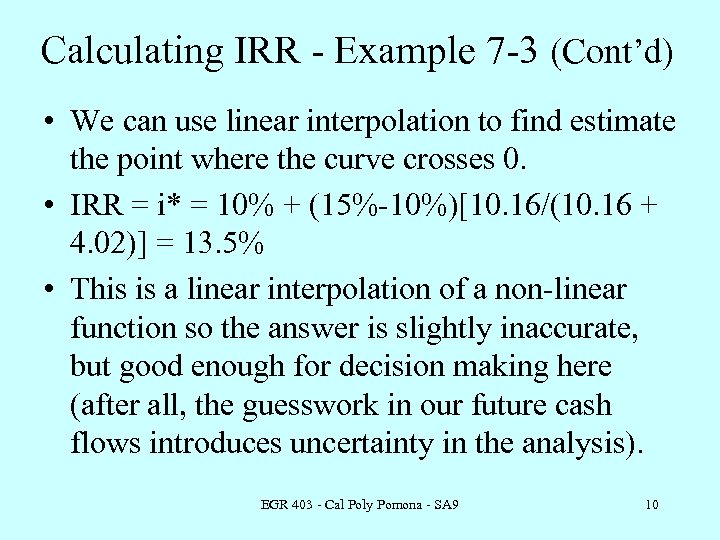Calculating IRR - Example 7 -3 (Cont’d) • We can use linear interpolation to find estimate the point where the curve crosses 0. • IRR = i* = 10% + (15%-10%)[10. 16/(10. 16 + 4. 02)] = 13. 5% • This is a linear interpolation of a non-linear function so the answer is slightly inaccurate, but good enough for decision making here (after all, the guesswork in our future cash flows introduces uncertainty in the analysis). EGR 403 - Cal Poly Pomona - SA 9 10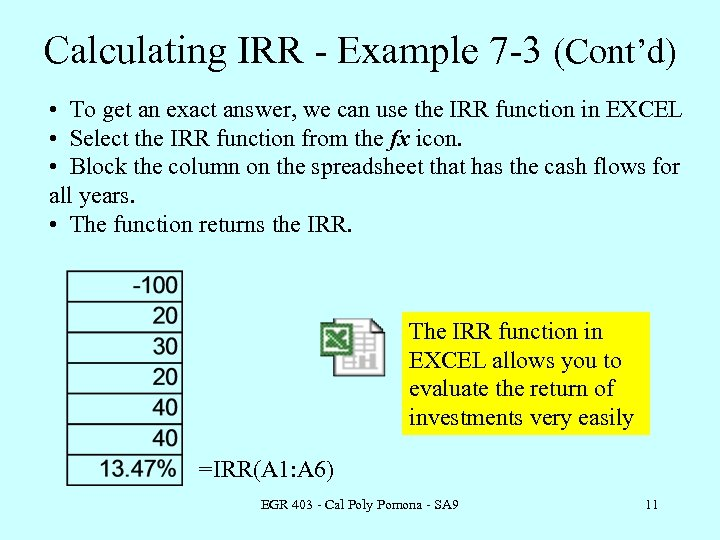Calculating IRR - Example 7 -3 (Cont’d) • To get an exact answer, we can use the IRR function in EXCEL • Select the IRR function from the fx icon. • Block the column on the spreadsheet that has the cash flows for all years. • The function returns the IRR. The IRR function in EXCEL allows you to evaluate the return of investments very easily =IRR(A 1: A 6) EGR 403 - Cal Poly Pomona - SA 9 11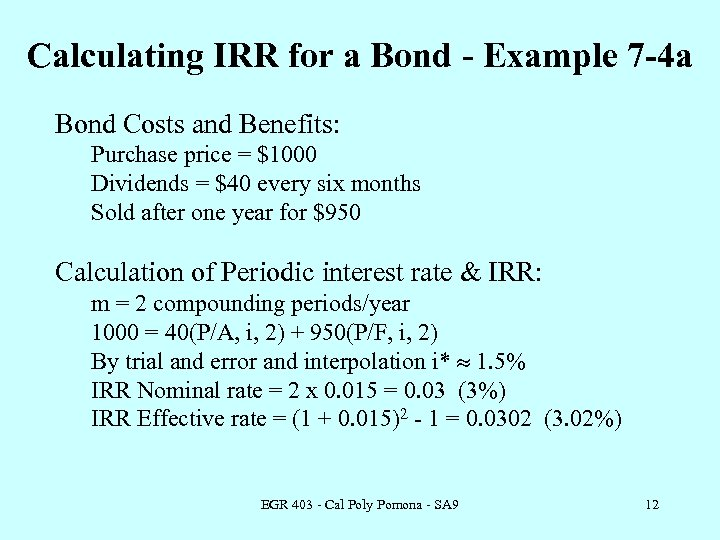Calculating IRR for a Bond - Example 7 -4 a Bond Costs and Benefits: Purchase price = \$1000 Dividends = \$40 every six months Sold after one year for \$950 Calculation of Periodic interest rate & IRR: m = 2 compounding periods/year 1000 = 40(P/A, i, 2) + 950(P/F, i, 2) By trial and error and interpolation i* » 1. 5% IRR Nominal rate = 2 x 0. 015 = 0. 03 (3%) IRR Effective rate = (1 + 0. 015)2 - 1 = 0. 0302 (3. 02%) EGR 403 - Cal Poly Pomona - SA 9 12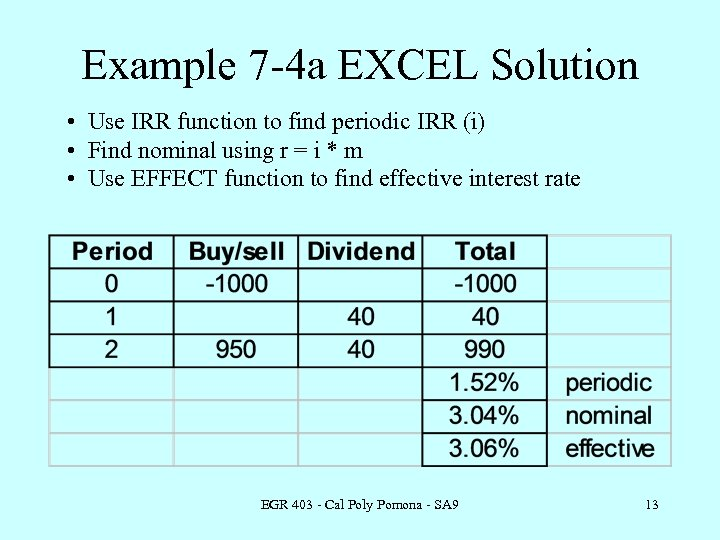Example 7 -4 a EXCEL Solution • Use IRR function to find periodic IRR (i) • Find nominal using r = i * m • Use EFFECT function to find effective interest rate EGR 403 - Cal Poly Pomona - SA 9 13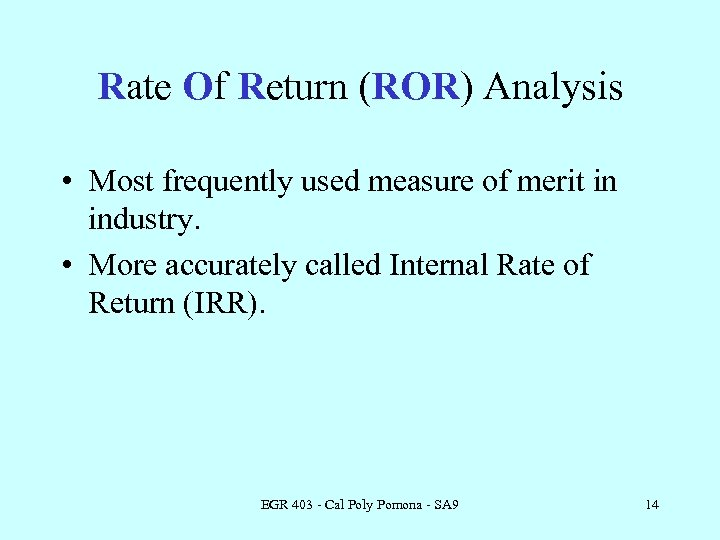Rate Of Return (ROR) Analysis • Most frequently used measure of merit in industry. • More accurately called Internal Rate of Return (IRR). EGR 403 - Cal Poly Pomona - SA 9 14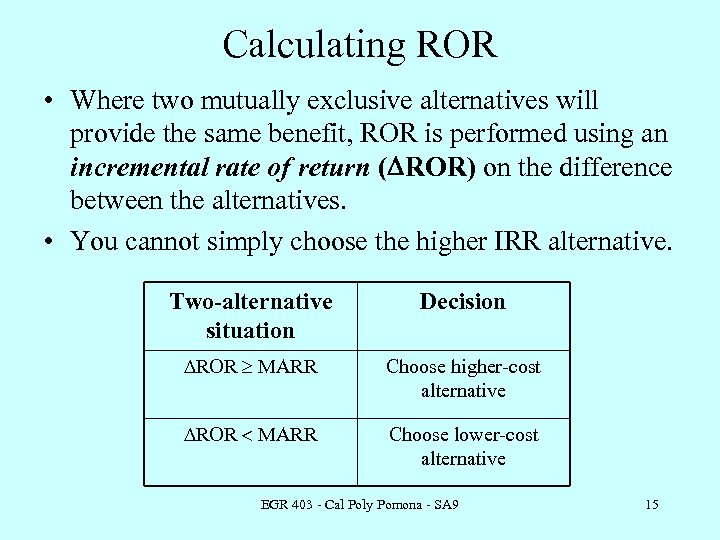Calculating ROR • Where two mutually exclusive alternatives will provide the same benefit, ROR is performed using an incremental rate of return (DROR) on the difference between the alternatives. • You cannot simply choose the higher IRR alternative. Two-alternative situation Decision DROR ³ MARR Choose higher-cost alternative DROR < MARR Choose lower-cost alternative EGR 403 - Cal Poly Pomona - SA 9 15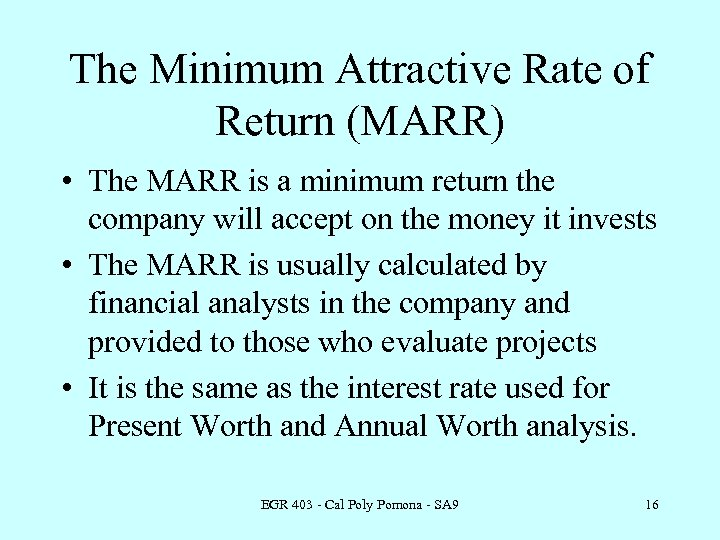The Minimum Attractive Rate of Return (MARR) • The MARR is a minimum return the company will accept on the money it invests • The MARR is usually calculated by financial analysts in the company and provided to those who evaluate projects • It is the same as the interest rate used for Present Worth and Annual Worth analysis. EGR 403 - Cal Poly Pomona - SA 9 16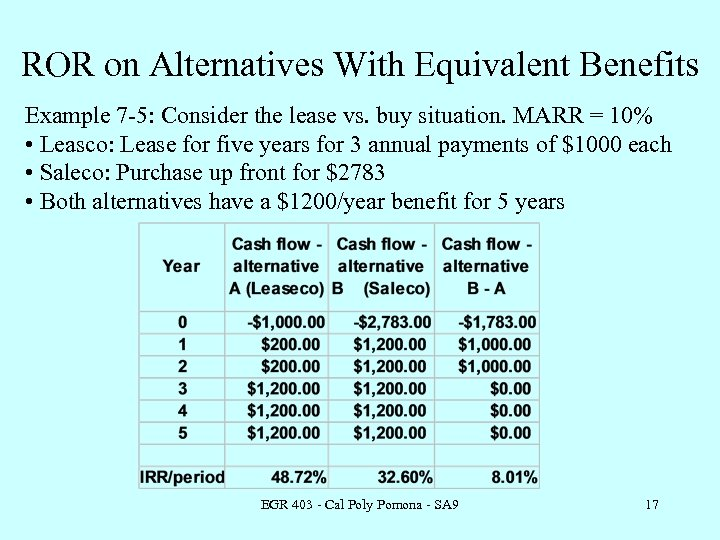ROR on Alternatives With Equivalent Benefits Example 7 -5: Consider the lease vs. buy situation. MARR = 10% • Leasco: Lease for five years for 3 annual payments of \$1000 each • Saleco: Purchase up front for \$2783 • Both alternatives have a \$1200/year benefit for 5 years EGR 403 - Cal Poly Pomona - SA 9 17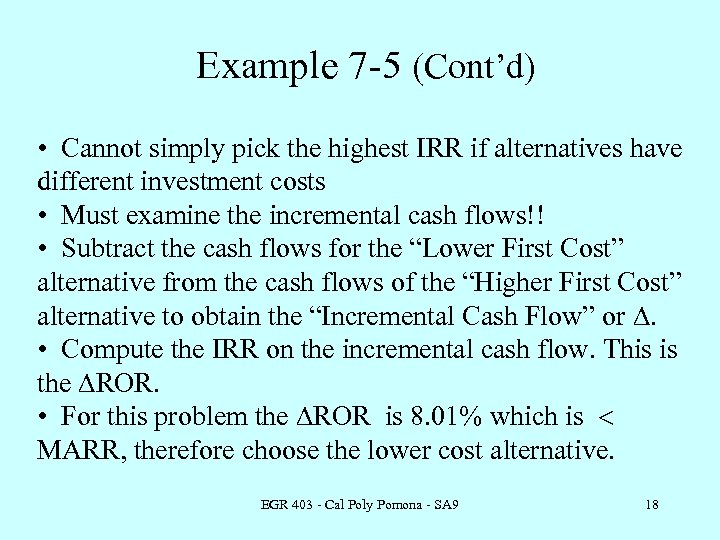Example 7 -5 (Cont’d) • Cannot simply pick the highest IRR if alternatives have different investment costs • Must examine the incremental cash flows!! • Subtract the cash flows for the “Lower First Cost” alternative from the cash flows of the “Higher First Cost” alternative to obtain the “Incremental Cash Flow” or D. • Compute the IRR on the incremental cash flow. This is the DROR. • For this problem the DROR is 8. 01% which is < MARR, therefore choose the lower cost alternative. EGR 403 - Cal Poly Pomona - SA 9 18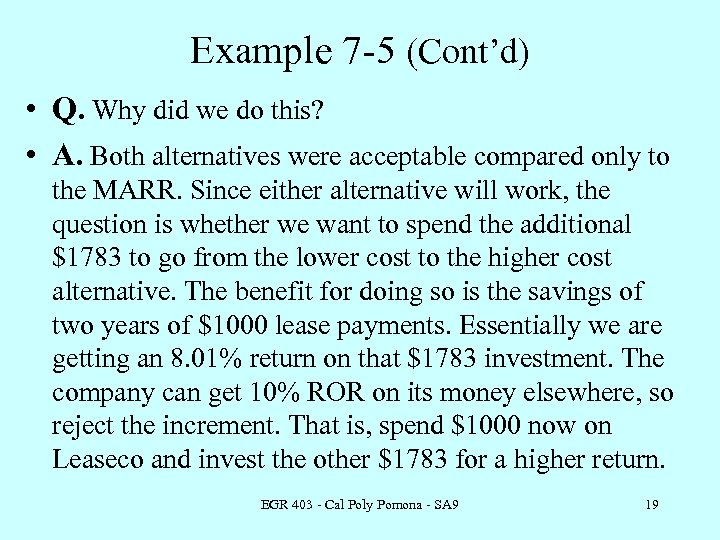Example 7 -5 (Cont’d) • Q. Why did we do this? • A. Both alternatives were acceptable compared only to the MARR. Since either alternative will work, the question is whether we want to spend the additional \$1783 to go from the lower cost to the higher cost alternative. The benefit for doing so is the savings of two years of \$1000 lease payments. Essentially we are getting an 8. 01% return on that \$1783 investment. The company can get 10% ROR on its money elsewhere, so reject the increment. That is, spend \$1000 now on Leaseco and invest the other \$1783 for a higher return. EGR 403 - Cal Poly Pomona - SA 9 19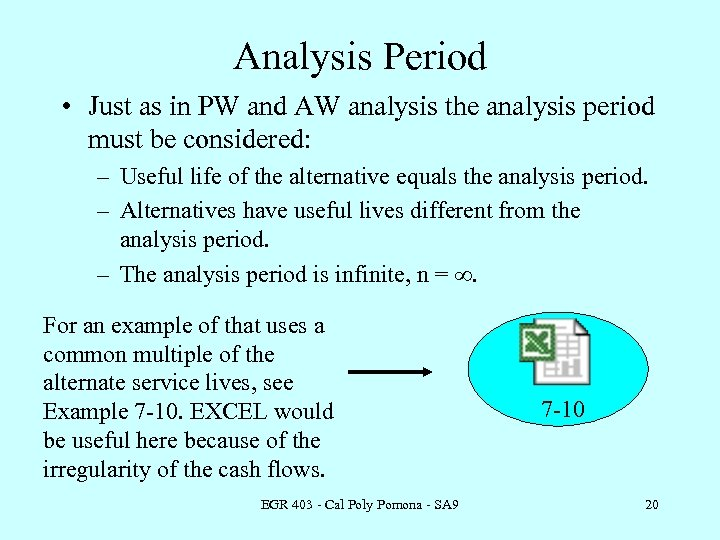Analysis Period • Just as in PW and AW analysis the analysis period must be considered: – Useful life of the alternative equals the analysis period. – Alternatives have useful lives different from the analysis period. – The analysis period is infinite, n = ¥. For an example of that uses a common multiple of the alternate service lives, see Example 7 -10. EXCEL would be useful here because of the irregularity of the cash flows. EGR 403 - Cal Poly Pomona - SA 9 7 -10 20